### Home > AC > Chapter 14 > Lesson 14.2.1.4 > Problem2-48

2-48.
1. In the diagram below, ∆ABC ~ ∆AED. Homework Help ✎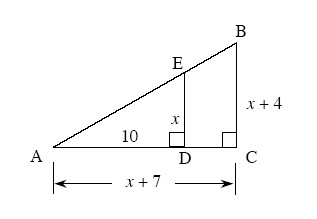1. Solve for x.

2. Find the perimeter of ∆ADE.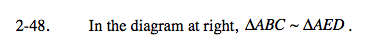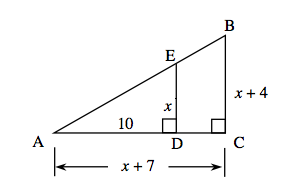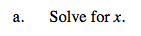The sides of the triangle are proportional. Write an equation and solve for x.

Because distance cannot be negative, x = 8, not x = −5.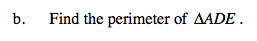Use your results from part (a) and the Pythagorean Theorem to determine the length of the hypotenuse.